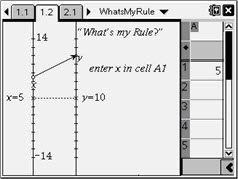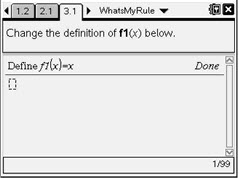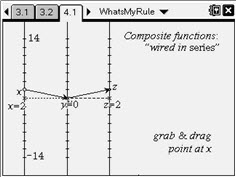# Activities

••• ##### Subject Area

• Math: Algebra II: Functions
• Math: Algebra II: Inverses
• Math: Algebra II: Radical Functions

• ##### Author9-12

20 Minutes

• ##### Device
• TI-Nspire™
• TI-Nspire™ CAS
• ##### Software

TI-Nspire™
TI-Nspire™ CAS

2.1

## What's My Rule?

#### Activity Overview

This activity is similar to a function machine. The nomograph is comprised of two vertical number lines, input on the left and output on the right. The transformation of input to output is illustrated dynamically by an arrow that connects a domain entry to its range value. Students try to find the rule of hidden functions by entering different domain values. They also use a nomograph with three vertical number lines to explore composite functions, including the composition of inverse functions.

#### Key Steps

•The first several problems are “What’s my Rule?” activities. Input values are entered, one at a time, into cell A1 of the spreadsheet on the right side of the screen. The nomograph on the left side of the screen displays the input and its corresponding output. By repeatedly entering different inputs, the student should be able to discover the function’s rule.

•Instruct students to create their own functions of the form y = ax + b or y = ax2 + b (where a and b are integers). Each student should use the Calculator work area on page 3.1 and redefine f1 to their own function by using the Recall Function Definition command.

•The nomograph in Problem 4 enables students to explore the meaning of the composition of functions.

Be sure students are familiar with both notations for composite functions: f2 ◦ f1 and f2(f1(x)).